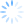The Start calculating after field becomes available when creating a price modifier with an overage action type. The value entered into this field specifies the starting point for calculations. Unlike the range, which determines when a price modifier should be used, the Start calculating after field determines when a price modifier should begin calculating.

This feature is especially useful if you wish to have an additional charges retroactively applied to orders when certain criteria are met. For example, if you wanted to create a price modifier that charged \$5 for every 10 miles over 100, you would use a range. However, if you wanted that price modifier to charge \$5 for every 10 miles above and below 100, but only if 100 miles is travelled, you would use the Start calculating after field. This way, if less than 100 miles were travelled, no additional charges would be added, but if more than 100 miles were travelled, OnTime would automatically go back and calculate a charge of \$5 for every 10 miles up to and after the 100.

Pingbacks and trackbacks (1)+